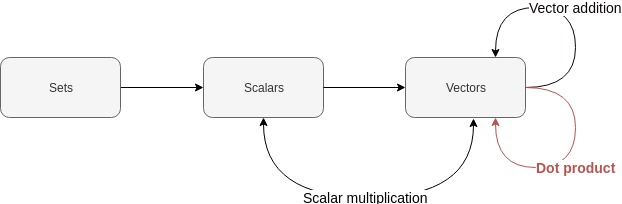Want to share your content on R-bloggers? click here if you have a blog, or here if you don't.

them element-wise. Sum the results. This is the dot product.)

LaTeX and MathJax warning for those viewing my feed: please view
directly on website!Hmmm…this is a tricky one!

Uhhh…did you know that Kendrick Lamar’s stage name used to be “K.Dot”?

Moi

Last
time
,
we learnt how to add vectors. It’s time to learn about dot products!# Today’s topic: dot products

Let’s define two vectors:

Let’s multiply these vectors element-wise. We’ll take the first
elements of our vectors and multiply them:

Let’s take the second elements and multiply them:

This, my friends, is the dot product of our vectors.

More generally, if we have an arbitrary vector $\boldsymbol{u}$ of
$n$ elements and another arbitrary vector $\boldsymbol{v}$ also of
$n$ elements, then the dot product $u \cdot v$ is:

The dot product $\boldsymbol{u} \cdot \boldsymbol{v}$ is equivalent to
$\boldsymbol{u}^T \boldsymbol{v}$. Let’s come back to this next time
when we talk about matrix multiplication.

## What is that angular ‘E’ looking thing?

For anyone who doesn’t know how to read the dot product equation, let’s
dissect its right-hand side!

$\sum$ is the uppercase form of the Greek letter ‘sigma’. In this
context, $\sum$ means ‘sum’. So we know that we’ll need to add some
things.

We have $\boldsymbol{u_i}$ and $\boldsymbol{v_i}$. In an earlier
post, we learnt that this refers to the $i$th element of some vector. So
we can refer to the first element of our vector $\boldsymbol{u}$ as
$u_1$. We notice that $v$ also shares the same subscript $i$. So
we know that whenever we refer to the second element in $u$ (i.e.
$u_2$), we will be referring to the second element in $v$ (i.e.
$v_2$).

We notice that $\boldsymbol{u_i}$ is next to $\boldsymbol{v_i}$. So
we’re going to be multiplying elements of our vectors which occur in the
same position, $i$.

We see that below our uppercase sigma there is a little $i=1$. We also
notice that there is a little $n$ above it. These mean “Let
$i = 1$. Keep incrementing $i$ until you reach $n$”.

What is $n$? It’s the number of elements in our vectors!

If we expand the right-hand side, we get:

This looks somewhat similar to the equation from the example earlier:

Easy! These are the mechanics of dot products.

## What the hell does this all mean anyway?

For a deeper understanding of dot products (which is unfortunately
beyond me right at this moment!) please refer to this video:

The entire series in the playlist is so beautifully done. They are
mesmerising!

# How can we perform dot products in R?

Let’s define two vectors:

x <- c(1, 2, 3)
y <- c(4, 5, 6)


We can find the dot product of these two vectors using the %*%
operator:

x %*% y

##      [,1]
## [1,]   32


What does R do if we simply multiply one vector by the other?

x * y

##   4 10 18


This is the element-wise product! If the dot product is simply the sum
of the element-wise product, then x %*% y is equivalent to doing this:

sum(x * y)

##  32


In our previous posts, R allowed us to multiply vectors of different
lengths. Notice how R doesn’t allow us to calculate the dot product of
vectors with different lengths:

x <- c(1, 2)
y <- c(3, 4, 5)

x %*% y


This is the exception that gets raised:

Error in x %*% y : non-conformable arguments

# Conclusion

We have learnt the mechanics of calculating dot products. We can now
finally move onto matrices. Ooooooh yeeeeeah.

Justin